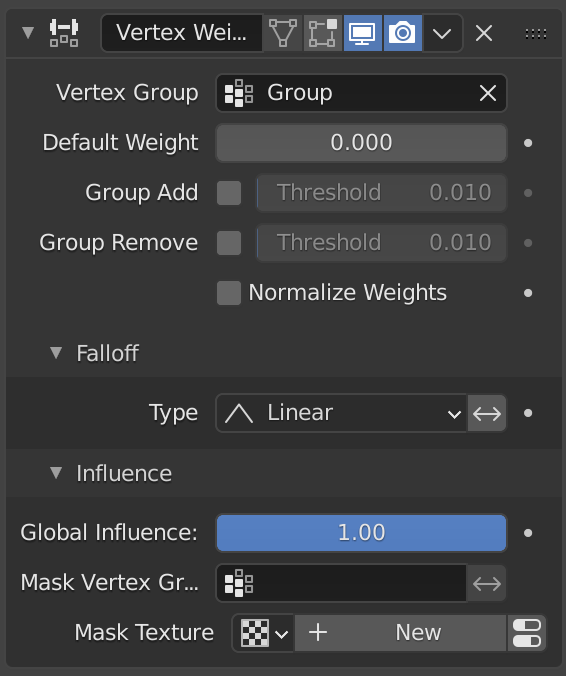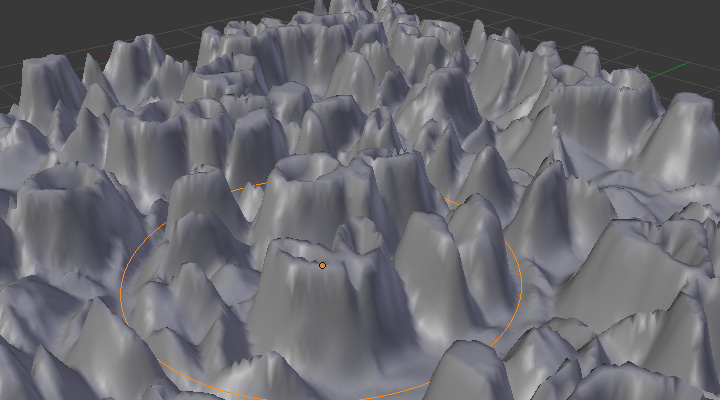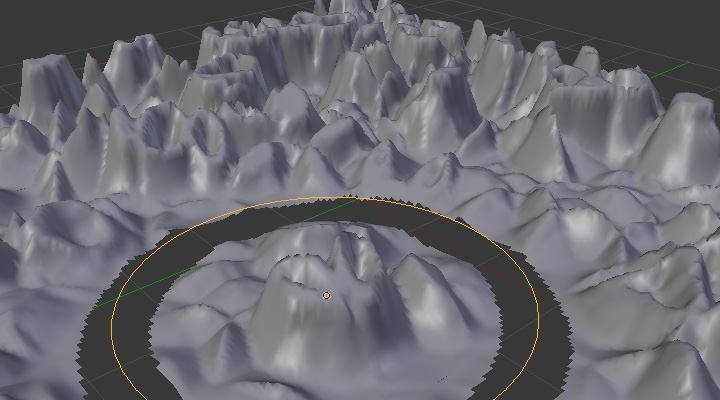# Vertex Weight Edit Modifier¶

This modifier is intended to edit the weights of a vertex group.

The general process is the following, for each vertex:

• (Optional) It does the mapping, either through one of the predefined functions, or a custom mapping curve.

• It applies the influence factor, and optionally the vertex group or texture mask (0.0 means original weight, 1.0 means fully mapped weight).

• It applies back the weight to the vertex, and/or it might optionally remove the vertex from the group if its weight is below a given threshold, or add it if it is above a given threshold.

Important

This modifier does implicit clamping of weight values in the standard (0.0 to 1.0) range. All values below 0.0 will be set to 0.0, and all values above 1.0 will be set to 1.0.

Note

You can view the modified weights in Weight Paint Mode. This also implies that you will have to disable the Vertex Weight Edit modifier if you want to see the original weights of the vertex group you are editing.

## Options¶The Vertex Weight Edit modifier panel.

Vertex Group

The vertex group to affect.

Default Weight

The default weight to assign to all vertices not in the given vertex group.

Adds vertices with a final weight over Add Threshold to the vertex group.

Group Remove

Removes vertices with a final weight below Remove Threshold from the vertex group.

Normalize Weights

Scale the weights in the vertex group to keep the relative weight but the lowest and highest values follow the full 0 - 1 range.

### Falloff¶

Falloff Type

Type of mapping.

Linear

No mapping.

Custom Curve

Allows you to manually define the mapping using a curve.

Sharp, Smooth, Root and Sphere

These are classical mapping functions, from spikiest to roundest.

Random

Uses a random value for each vertex.

Median Step

Creates binary weights (0.0 or 1.0), with 0.5 as cutting value.

Invert <-->

Inverts the falloff.

### Influence¶

Those settings are the same for the three Vertex Weight modifiers.

Global Influence

The overall influence of the modifier (0.0 will leave the vertex group’s weights untouched, 1.0 is standard influence).

Important

Influence only affects weights, adding/removing of vertices to/from vertex group is not prevented by setting this value to 0.0.

In addition, a per-vertex fine control of the effect is possible using either a vertex group or a texture (both are mutually exclusive). The per-vertex values from those will be multiplied with the Global Influence.

See common masking options for a complete reference.

## Example¶

Here is an example of various effects achieved using Vertex Weight Edit modifier (together with the Vertex Weight Proximity modifier) to generate weights used by the Displace modifier.Concave-type mapping curve.¶Vertices with a computed weight below 0.1 removed from the vertex group.

The blend-file, TEST_2 scene.